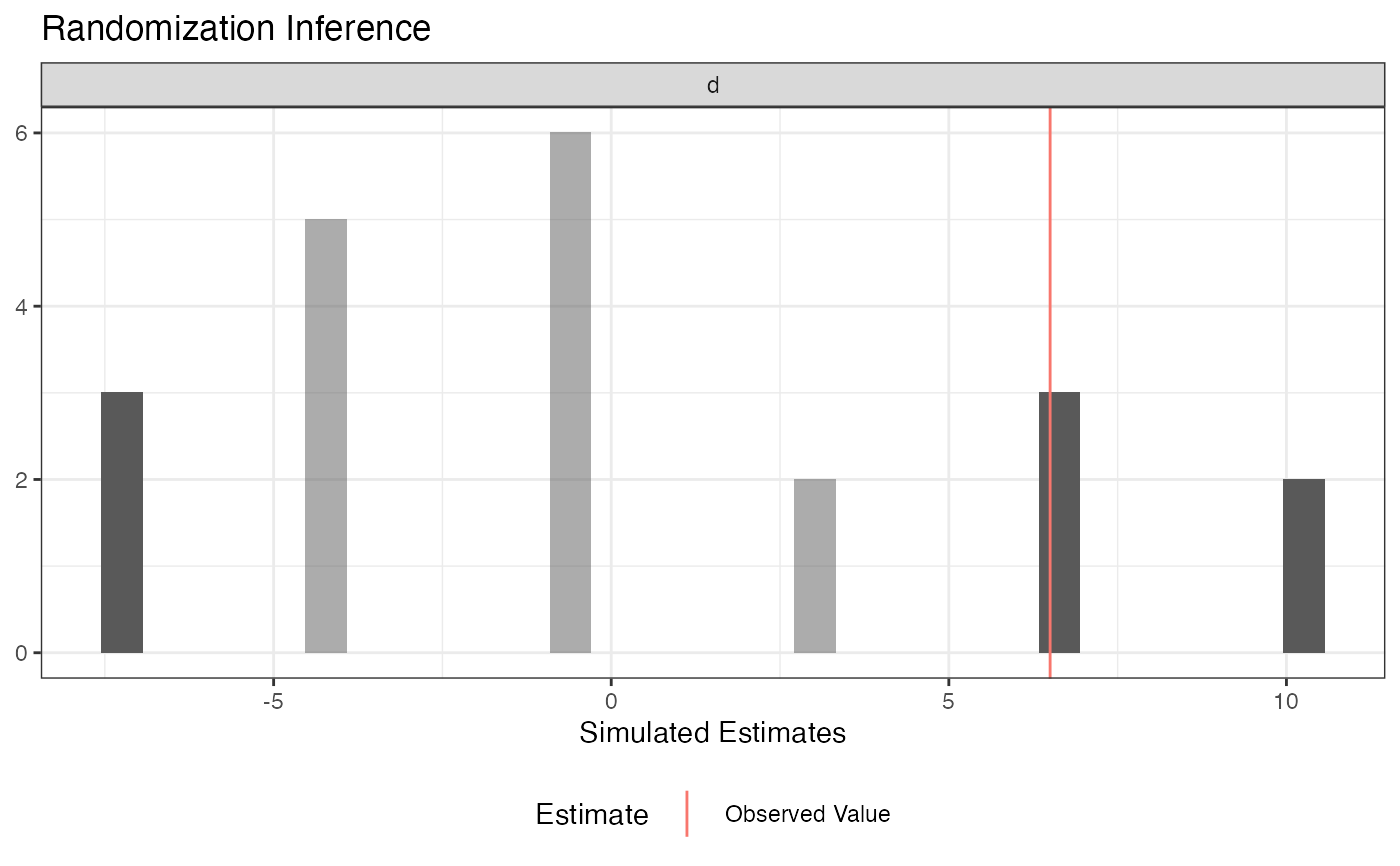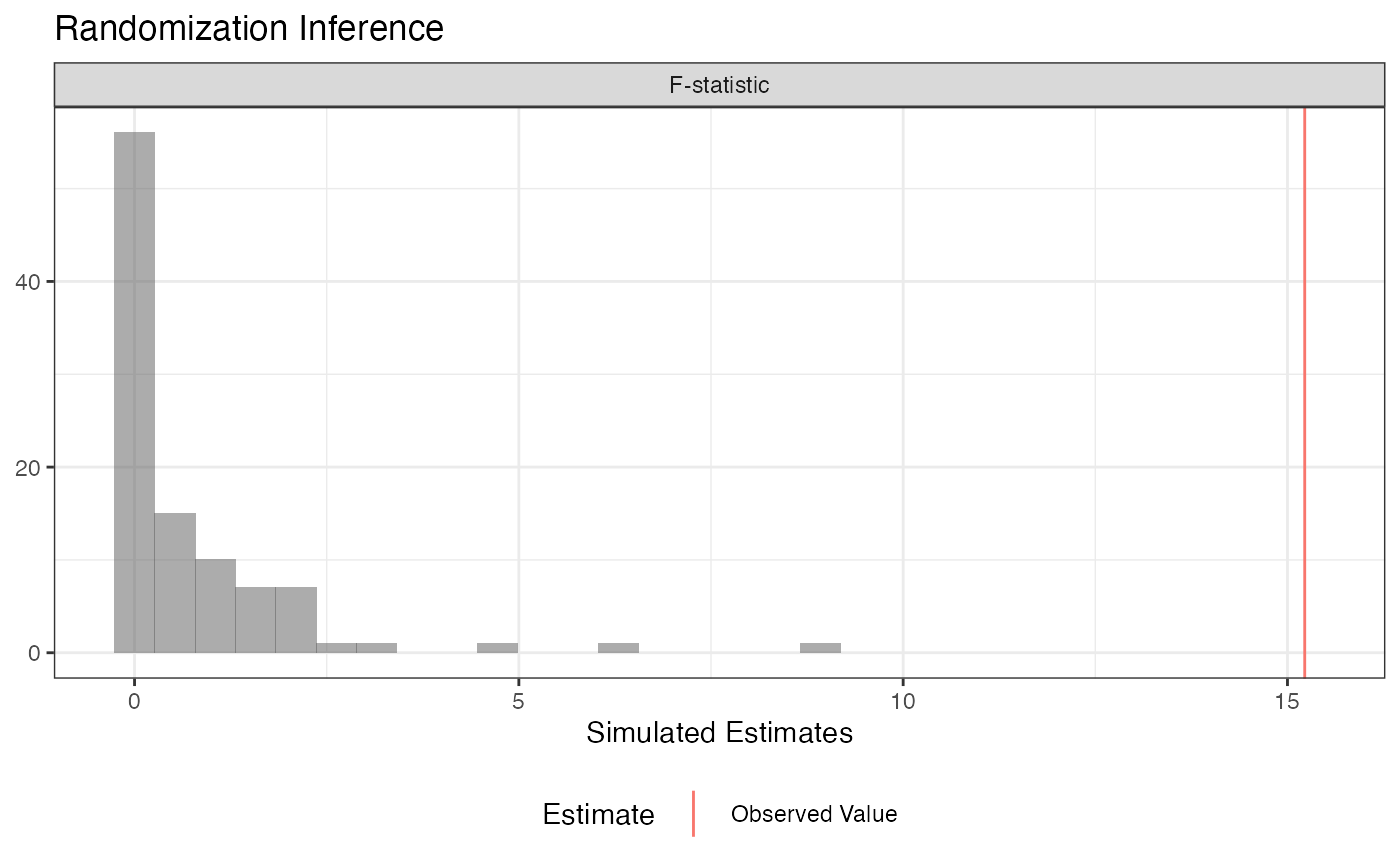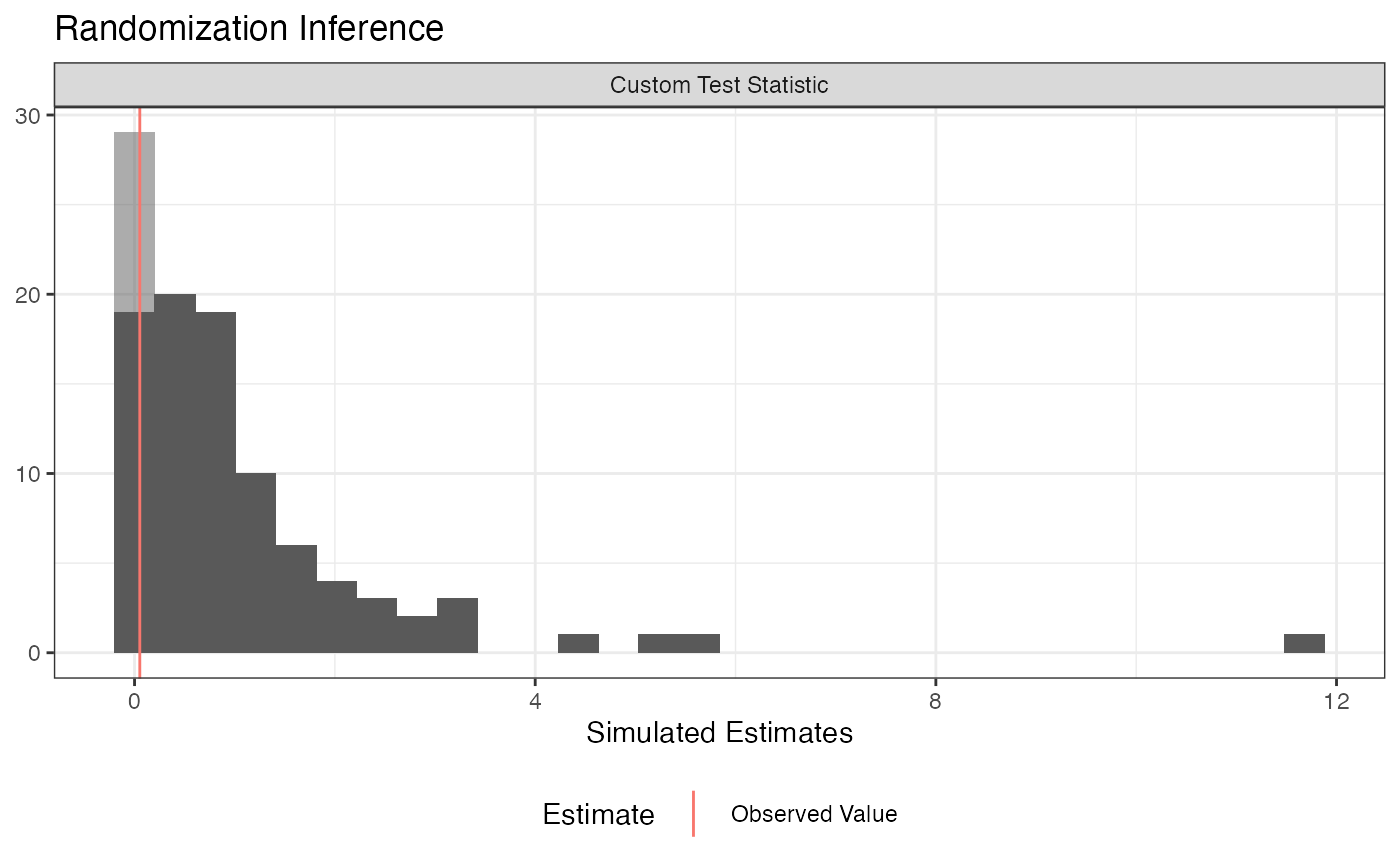This function makes it easy to conduct three kinds of randomization inference.

conduct_ri(
formula = NULL,
model_1 = NULL,
model_2 = NULL,
test_function = NULL,
assignment = "Z",
outcome = NULL,
declaration = NULL,
sharp_hypothesis = 0,
studentize = FALSE,
IPW = TRUE,
IPW_weights = NULL,
sampling_weights = NULL,
permutation_matrix = NULL,
data,
sims = 1000,
progress_bar = FALSE,
p = "two-tailed"
)

## Arguments

formula an object of class formula, as in lm. Use formula when conducting significance tests of an Average Treatment Effect estimate under a sharp null hypothesis. For the difference-in-means estimate, do not include covariates. For the OLS covariate-adjusted estimate, include covariates. an object of class formula, as in lm. Models 1 and 2 must be "nested." model_1 should be the "restricted" model and model_2 should be the "unrestricted" model. an object of class formula, as in lm. Models 1 and 2 must be "nested." model_1 should be the "restricted" model and model_2 should be the "unrestricted" model. A function that takes data and returns a scalar test statistic. a character string that indicates which variable is randomly assigned. Defaults to "Z". a character string that indicates which variable is the outcome variable. Defaults to NULL. A random assignment declaration, created by declare_ra. either a numeric scalar or a numeric vector of length k - 1, where k is the number of treatment conditions. In a two-arm trial, this number is the *hypothesized* difference between the treated and untreated potential potential outcomes for each unit.. In a multi-arm trial, each number in the vector is the hypothesized difference in potential outcomes between the baseline condition and each successive treatment condition. logical, defaults to FALSE. Should the test statistic be the t-ratio rather than the estimated ATE? T-ratios will be calculated using HC2 robust standard errors or their clustered equivalent. CLUSTERING NOT YET IMPLEMENTED. logical, defaults to TRUE. Should inverse probability weights be calculated? a character string that indicates which variable is the existing inverse probability weights vector. Usually unnecessary, as IPW weights will be incorporated automatically if IPW = TRUE. Defaults to NULL. a character string that indicates which variable is the sampling weights vector. Optional, defaults to NULL. NOT YET IMPLEMENTED An optional matrix of random assignments, typically created by obtain_permutation_matrix. A data.frame. the number of simulations. Defaults to 1000. logical, defaults to FALSE. Should a progress bar be displayed in the console? Should "two-tailed", "upper", or "lower" p-values be reported? Defaults to "two-tailed". For two-tailed p-values, whether or not a simulated value is as large or larger than the observed value is determined with respect to the distance to the sharp null.

## Details

1. Conduct hypothesis tests under the sharp null when the test statistic is the difference-in-means or covariate-adjusted average treatment effect estimate. 2. Conduct "ANOVA" style hypothesis tests, where the f-statistic from two nested models is the test statistic. This procedure is especially helpful when testing interaction terms under null of constant effects. 3. Arbitrary (scalar) test statistics

## Examples


# Data from Gerber and Green Table 2.2

# Randomization Inference for the Average Treatment Effect

table_2.2 <-
data.frame(d = c(1, 0, 0, 0, 0, 0, 1),
y = c(15, 15, 20, 20, 10, 15, 30))

## Declare randomization procedure
declaration <- declare_ra(N = 7, m = 2)

## Conduct Randomization Inference
out <- conduct_ri(y ~ d,
declaration = declaration,
assignment = "d",
sharp_hypothesis = 0,
data = table_2.2)

summary(out)
#>   term estimate two_tailed_p_value
#> 1    d      6.5          0.3809524plot(out)tidy(out)
#>   term estimate   p.value
#> 1    d      6.5 0.3809524
# Using a custom permutation matrix

permutation_matrix <-
matrix(c(0, 0, 0, 0, 0, 0, 1,
0, 0, 0, 0, 0, 1, 0,
0, 0, 0, 0, 1, 0, 0,
0, 0, 0, 1, 0, 0, 0,
0, 0, 1, 0, 0, 0, 0,
0, 1, 0, 0, 0, 0, 0,
1, 0, 0, 0, 0, 0, 0),
ncol = 7)

conduct_ri(y ~d, assignment = "d", data = table_2.2,
permutation_matrix = permutation_matrix)
#>   term estimate two_tailed_p_value
#> 1    d      6.5          0.2857143

# Randomization Inference for an Interaction

N <- 100
declaration <- randomizr::declare_ra(N = N, m = 50)

Z <- randomizr::conduct_ra(declaration)
X <- rnorm(N)
Y <- .9 * X + .2 * Z + 1 * X * Z + rnorm(N)
dat <- data.frame(Y, X, Z)

ate_obs <- coef(lm(Y ~ Z, data = dat))

out <-
conduct_ri(
model_1 = Y ~ Z + X,
model_2 = Y ~ Z + X + Z * X,
declaration = declaration,
assignment = "Z",
sharp_hypothesis = ate_obs,
data = dat, sims = 100
)

plot(out)summary(out)
#>          term estimate two_tailed_p_value
#> 1 F-statistic 15.22457                  0
summary(out, p = "two-tailed")
#>          term estimate two_tailed_p_value
#> 1 F-statistic 15.22457                  0summary(out, p = "upper")
#>          term estimate upper_p_value
#> 1 F-statistic 15.22457             0summary(out, p = "lower")
#>          term estimate lower_p_value
#> 1 F-statistic 15.22457             1
tidy(out)
#>          term estimate p.value
#> 1 F-statistic 15.22457       0
# Randomization Inference for arbitrary test statistics

## In this example we're conducting a randomization check (in this case, a balance test).

N <- 100
declaration <- randomizr::declare_ra(N = N, m = 50)

Z <- randomizr::conduct_ra(declaration)
X <- rnorm(N)
Y <- .9 * X + .2 * Z + rnorm(N)
dat <- data.frame(Y, X, Z)

balance_fun <- function(data) {
f_stat <- summary(lm(Z ~ X, data = data))\$f
names(f_stat) <- NULL
return(f_stat)
}

## confirm function works as expected
balance_fun(dat)
#>  0.05245928
## conduct randomization inference

out <-
conduct_ri(
test_function = balance_fun,
declaration = declaration,
assignment = "Z",
sharp_hypothesis = 0,
data = dat, sims = 100
)

plot(out)summary(out)
#>                    term   estimate two_tailed_p_value
#> 1 Custom Test Statistic 0.05245928                0.9tidy(out)
#>                    term   estimate p.value
#> 1 Custom Test Statistic 0.05245928     0.9